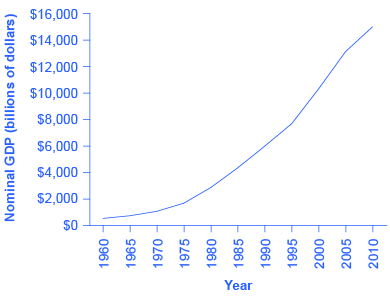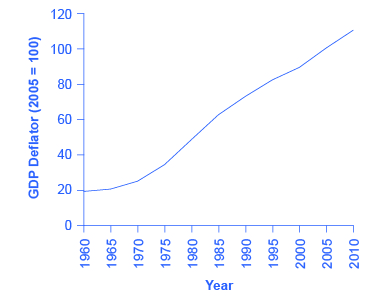# 19.2 Adjusting nominal values to real values

 Page 1 / 9

By the end of this section, you will be able to:

• Contrast nominal GDP and real GDP
• Explain GDP deflator
• Calculate real GDP based on nominal GDP values

When examining economic statistics, there is a crucial distinction worth emphasizing. The distinction is between nominal and real measurements, which refer to whether or not inflation has distorted a given statistic. Looking at economic statistics without considering inflation is like looking through a pair of binoculars and trying to guess how close something is: unless you know how strong the lenses are, you cannot guess the distance very accurately. Similarly, if you do not know the rate of inflation, it is difficult to figure out if a rise in GDP is due mainly to a rise in the overall level of prices or to a rise in quantities of goods produced. The nominal value    of any economic statistic means the statistic is measured in terms of actual prices that exist at the time. The real value    refers to the same statistic after it has been adjusted for inflation. Generally, it is the real value that is more important.

## Converting nominal to real gdp

[link] shows U.S. GDP at five-year intervals since 1960 in nominal dollars; that is, GDP measured using the actual market prices prevailing in each stated year. This data is also reflected in the graph shown in [link]

U.s. nominal gdp and the gdp deflator
Year Nominal GDP (billions of dollars) GDP Deflator (2005 = 100)
1960 543.3 19.0
1965 743.7 20.3
1970 1,075.9 24.8
1975 1,688.9 34.1
1980 2,862.5 48.3
1985 4,346.7 62.3
1990 5,979.6 72.7
1995 7,664.0 81.7
2000 10,289.7 89.0
2005 13,095.4 100.0
2010 14,958.3 110.0Nominal GDP values have risen exponentially from 1960 through 2010, according to the BEA.

If an unwary analyst compared nominal GDP in 1960 to nominal GDP in 2010, it might appear that national output had risen by a factor of twenty-seven over this time (that is, GDP of \$14,958 billion in 2010 divided by GDP of \$543 billion in 1960). This conclusion would be highly misleading. Recall that nominal GDP is defined as the quantity of every good or service produced multiplied by the price at which it was sold, summed up for all goods and services. In order to see how much production has actually increased, we need to extract the effects of higher prices on nominal GDP. This can be easily done, using the GDP deflator.

GDP deflator is a price index measuring the average prices of all goods and services included in the economy. We explore price indices in detail and how they are computed in Inflation , but this definition will do in the context of this chapter. The data for the GDP deflator are given in [link] and shown graphically in [link] .Much like nominal GDP, the GDP deflator has risen exponentially from 1960 through 2010. (Source: BEA)

[link] shows that the price level has risen dramatically since 1960. The price level in 2010 was almost six times higher than in 1960 (the deflator for 2010 was 110 versus a level of 19 in 1960). Clearly, much of the apparent growth in nominal GDP was due to inflation, not an actual change in the quantity of goods and services produced, in other words, not in real GDP. Recall that nominal GDP can rise for two reasons: an increase in output, and/or an increase in prices. What is needed is to extract the increase in prices from nominal GDP so as to measure only changes in output. After all, the dollars used to measure nominal GDP in 1960 are worth more than the inflated dollars of 1990—and the price index tells exactly how much more. This adjustment is easy to do if you understand that nominal measurements are in value terms, where

What is the law of demand
price increase demand decrease...price decrease demand increase
Mujahid
ıf the price increase the demand decrease and if the demand increase the price decrease
MUBARAK
all other things being equal, an increase in demand causes a decrease in supply and vice versa
SETHUAH
yah
Johnson
how is the economy of usa now
Johnson
What is demand
Demand is the quantity of goods and services a consumer is willing and able to purchase at various prices over a given period of time.
Yaw
yea
SETHUAH
Okay congratulations I'll join you guys later .
Aj
yes
MUBARAK
calculate elasticity of income exercises
If potatoes cost Jane \$1 per kilogram and she has \$5 that could possibly spend on potatoes or other items. If she feels that the first kilogram of potatoes is worth \$1.50, the second kilogram is worth\$1.14, the third is worth \$1.05 and subsequent kilograms are worth \$0.30, how many kilograms of potatoes will she purchase? What if she only had \$2 to spend?
cause of poverty in urban
QI: (A) Asume the following cost data are for a purely competitive producer: At a product price Of \$56. will this firm produce in the short run? Why Why not? If it is preferable to produce, what will be the profit-maximizing Or loss-minimizing Output? Explain. What economic profit or loss will the
what is money
what is economic
economics is the study of ways in which people use resources to satisfy their wants
Falak
what is Price mechanism
introduction to economics
welfare definition of economics
Uday
examine the wealth and welfare definitions of economics
Uday
Anand
What do we mean by Asian tigers
Dm me I will tell u
Shailendra
Hi
Aeesha
hi
Pixel
What is Average revenue
KEMZO
How are u doing
KEMZO
it is so fantastic
metasebia
uday
Uday
it is a group of 4 countries named Singapore, South Korea, Taiwan and Hong Kong because their economies are growing very faster
Anand
fyn
EDWARD
Please, average revenue is an amount of money you gained after deducted your total expenditure from your total income.
EDWARD
what's a demand
it is the quantity of commodities that consumers are willing and able to purchase at particular prices and at a given time
Munanag
quantity of commodities dgat consumers are willing to pat at particular price
Omed
demand depends upon 2 things 1wish to buy 2 have purchasing power of that deserving commodity except any from both can't be said demand.
Bashir
Demand is a various quantity of a commodities that a consumer is willing and able to buy at a particular price within a given period of time. All other things been equal.
Vedzi
State the law of demand
Vedzi
The desire to get something is called demand.
Mahabuba
what is the use of something should pay for its opportunity foregone to indicate?
Why in monopoly does the firm maximize profits when its marginal revenue equals marginal cost
different between economic n history

#### Get Jobilize Job Search Mobile App in your pocket Now!ByBy Naveen TomarBy Megan EarhartBy David GeltnerBy OpenStaxBy Bonnie HurstBy John GabrieliBy RhodesBy Janet ForresterBy Anh DaoBy Jazzycazz Jackson